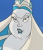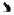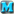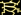<< Back to Off-topic Forum .GrayedOutTextBox { color: Gray; }

 Posts 1 - 7 of 7
Finding the derivative of a function: 10/2/2019 02:26:25𝘝𝘌𝘙𝘕𝘈𝘓 𝘝𝘐𝘕𝘈𝘐𝘎𝘙𝘌𝘛𝘛𝘌
Level 38
Report
f(t) = 8(t^4 − 6)^5

find f'(t)

How is this incorrect:

8(5)(t^4-6)^4 - general power law?
Finding the derivative of a function: 10/2/2019 02:30:52𝘝𝘌𝘙𝘕𝘈𝘓 𝘝𝘐𝘕𝘈𝘐𝘎𝘙𝘌𝘛𝘛𝘌
Level 38
Report
SOLVED:

40\left(t^4-6\right)^4\left(4t^{\left(3\right)}\right)
Finding the derivative of a function: 10/2/2019 02:40:25𝘝𝘌𝘙𝘕𝘈𝘓 𝘝𝘐𝘕𝘈𝘐𝘎𝘙𝘌𝘛𝘛𝘌
Level 38
Report
f(x)=((x+8)/(x-8))^5

f'(x)=5((x+8)/x-8))^4

how is this wrong?

edit: figured out about the quotient rule but somehow this is still wrong

5\left(\frac{x+8}{x-8}\right)^4\left(\frac{\left(x-8\right)-\left(x+8\right)}{\left(x+8\right)^2}\right)

wtf?

Edited 10/2/2019 02:50:25
Finding the derivative of a function: 10/2/2019 02:49:09Emperor Cacao
Level 56
Report
I'm pretty sure you were supposed to take as

(x + 8)^5
__________
(x - 8)^5

you have a variable in both sides of the division bar
Finding the derivative of a function: 10/2/2019 04:13:49JustinR17Level 58
Report
Finding the derivative of a function: 10/2/2019 04:52:29𝘝𝘌𝘙𝘕𝘈𝘓 𝘝𝘐𝘕𝘈𝘐𝘎𝘙𝘌𝘛𝘛𝘌
Level 38
Report
Thanks dude, but I already submitted the online homework.
Finding the derivative of a function: 10/2/2019 13:51:38Farah♦Level 60
Report
Whenever you have a function f(g(x)), you take the derivative of f(g(x)) while considering g(x) as a constant and multiply that by the derivative of g(x). So d/dx[f(g(x))] = f'(g(x)) · g'(x)
 Posts 1 - 7 of 7